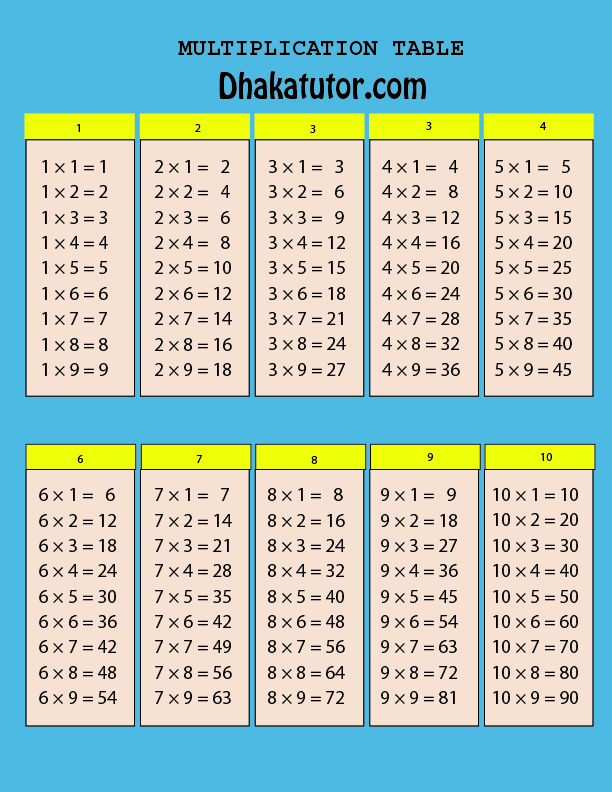# Multiplication Table | Multiplication Chart

Published on 05-May-2023

# Multiplication Calculator

## Multiplication table

A multiplication table is a mathematical table used to define the results of multiplication operation between two integers. It is a table that displays the products of all the possible combinations of numbers from 1 to 10 or 12, depending on the table's design.

The multiplication table consists of a grid where the first row and column contain the numbers 1 to 10 or 12. Each cell in the table represents the product of the corresponding row and column numbers. For example, the cell in the second row and third column represents the product of 2 and 3, which is 6.

Multiplication tables are commonly used in mathematics education to help students learn and memorize multiplication facts. By memorizing the multiplication table, students can quickly and accurately solve multiplication problems without the need for additional calculations.

While there are various methods to memorize the multiplication table, repetition and practice are generally considered to be the most effective. With regular practice and review, students can quickly master the multiplication table and develop a strong foundation in mathematics.## Multiplication Chart### More Article

Tag  #